# NEET UG Physics Work Energy MCQs

NEET UG Physics Work Energy MCQs with answers available in Pdf for free download. The MCQ Questions for NEET Physics with answers have been prepared as per the latest NEET Physics syllabus, books and examination pattern. Multiple Choice Questions form important part of competitive exams and NEET exam and if practiced properly can help you to get higher rank. Refer to more topic wise NEET Physics Questions and also download more latest study material for all subjects and do free NEET Physics Mock Test

## Work Energy NEET Physics MCQ

NEET Physics students should refer to the following multiple-choice questions with answers for Work Energy in NEET. These MCQ questions with answers for NEET Physics will come in exams and help you to score good marks

### Work Energy MCQ Questions with Answers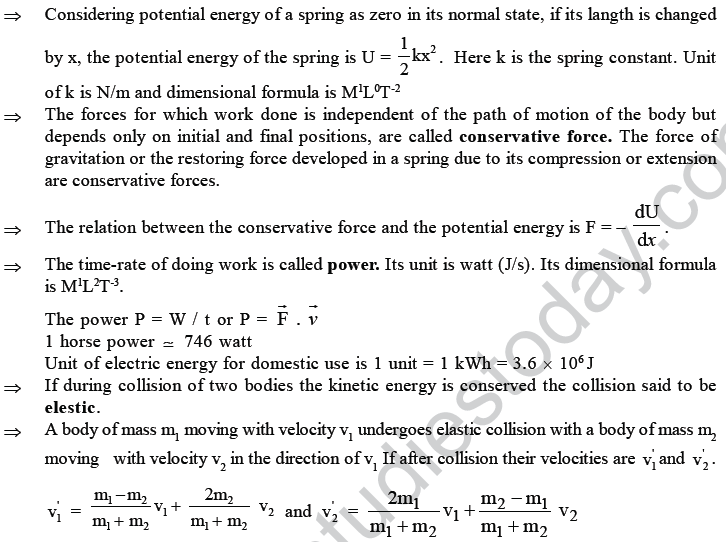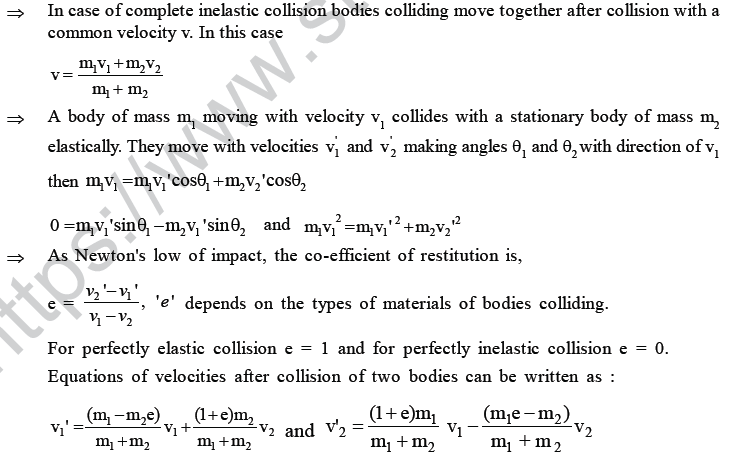For the answer of the following questions choose the correct alternative from among the given ones.

1. How much is the work done in pulling up a block of wood weighing 2KN for a length of 10m on a smooth plane inclined at an angle of 30o with the horizontal ?
(A) 1.732 KJ (B) 17.32 KJ (C) 10 KJ (D) 100 KJ

2. A force of 7N, making an angle θ with the horizontal, acting on an object displaces it by 0.5m along the horizontal direction. If the object gains K.E. of 2J, what is the horizontal component of the force ?
(A) 2N (B) 4 N (C) 1 N (D) 14 N

3. A 60 kg JATAN with 10 kg load on his head climbs 25 steps of 0.20m height each. what is the work done in climbing ? (g = 10 m/s2)
(A) 5 J (B) 350 J (C) 100J (D) 3500J

4. A ball of mass 5 kg is stiding on a plane with intial velocity of 10 m/s. If coefficient of friction between surface and ball is 1/2 , then before stopping it will describe...... (g = 10 m/s2)

(A) 12.5 m (B) 5 m (C) 7.5 m (D) 10 m

5. The relationship between force and position is shown in the figure given (in one dimensional case) calculate the work done by the force in displacing a body from x = 0 cm to x = 5 cm
(A) 30 ergs (B) 70 ergs (C) 20 ergs (D) 60 ergs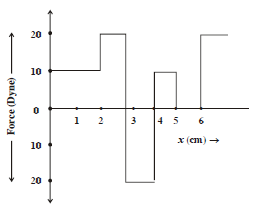6. The force constant of a wire is K and that of the another wire is 3k when both thewires are stretched through same distance, if work done are W1 and W2, then...
(A) w= 3w12 (B) W2 = 0.33W1 (C) W2 = W1 (D) W2 = 3W1

7. A ball is released from the top of a tower. what is the ratio of work done by force of gravity in first, second and third second of the motion of the ball ? [hn α (2n – 1)]
(A) 1 : 2 : 3 (B) 1 : 4 : 9 (C) 1 : 3 : 5 (D) 1: 5 : 3

8. A spring of spring constant 103 N/m is stretched initially 4cm from the unstretched position. How much the work required to stretched it further by another 5 cm ?

(A) 6.5 NM (B) 2.5 NM (C) 3.25 NM (D) 6.75 NM

9. The mass of a car is 1000 kg. How much work is required to be done on it to make it move with a speed of 36 km/h ?
(A) 2.5×104 J (B) 5×103 J (C) 500 J (D) 5×104 J

10. A body of mass 6 kg is under a force, which causes a displacement in it given by S = 2t3 /3 (in m). Find the work done by the force in first one seconds.

(A) 2 J (B) 3.8 J (C) 5.2 J (D) 24 J

11. A 8 kg mass moves along x - axis. Its accelerations as a function of its position is shown in the figure. What is the total work done on the mass by the force as the mass moves from x = 0 to x = 6cm ?
(A) 48×10-3 J (B) 98× 10-3 J (C) 4.8×10-3 J (D) 9.8×10-3 J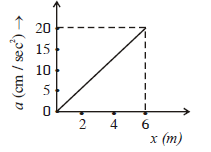12. The work done by a force acting on a body is as shown in the graph.what is the total work done in covering an intial distance of 15m ?
(A) 50 J (B) 75 J (C) 100 J (D) 25 J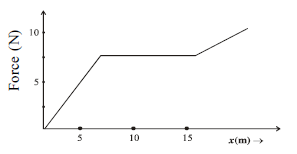13. A spring gun of spring constant 90×102N /M is compressed 4cm by a ball of mass 16g. If the trigger is pulled, calculate the velocity of the ball.
(A) 60 m/s (B) 3 m/s (C) 90 m/s (D) 30 m/s

14. A uniform chain of length 2m is kept on a table such that a length of 50cm hangs freely from the edge of the table. The total mass of the chain is 5kg. What is the work done in pulling the entire chain on the table. (g = 10 m\s2)
(A) 7.2 J (B) 3 J (C) 4.6 J (D) 120 J

15. A uniform chain of length L ans mass M is lying on a smooth table and one third of its is hanging vertically down over the edge of the table. If g is acceleration due to gravity, the work required to pull the hanging part on to the table is
(A) MgL (B) MgL / 3 (C) MgL/9 (D) MgL / 18

16. A cord is used to lower vertically a block of mass M by a distance d with constant down-word acceleration 9/2 .work done by the cord on the block is
(A) - Mgd/2 (B) Mgd/4 (C) -3Mgd/4 (D) Mgd

17. A block of mass 5 kg is resting on a smooth surface. At what angle a force of 20N be acted on the body so that it will acquired a kinetic energy of 40J after moving 4m
(A) 30° (B) 45° (C) 60°(D) 120°

18. Natural length of a spring is 60 cm, and its spring constant is 2000N/m. A mass of 20kg is hung from it. The extension produced in the spring is ____ (g = 9.8 m/s2)
(A) 4.9 cm (B) 0.49 cm (C) 9.8 cm (D) 0.98 cm

19. The potential energy of a body is given by U = A - Bx2 (where x is displacement).
The magnitude of force acting on the particle is
(A) constant (B) proportional to x
(C) proportional to x2 (D) Inversely proportional to x

20. A uniform chain of length L and mass M is lying on a smooth table and (1/ 4 )th of its length is hanging vertically down over the edge of the table. If g is acceleration due to gravity, the work required to pull the hanging part on to the table is
(A) MgL (B) MgL/9 (C) MgL/18 (D) MgL/ 32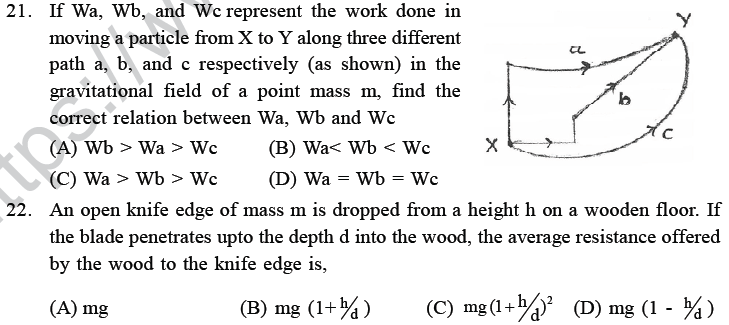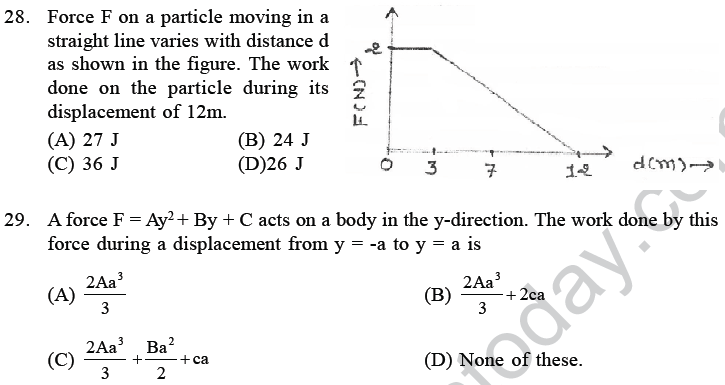(ENERGY AND CONSERVATVE, NON CONSERVATIVE FORCE)

30. A spring with spring constant K when streched through 2cm the potential energy is U. If it is streched by 6cm. The potential energy will be _____
(A) 6U (B) 3U (C) 9U (D) 18U

31. If linear momentum of body is increased by 1.5%, its kinetic energy increases by ______
(A) 0% (B) 10% (C) 2.25% (D) 3%

32. With what velocity should a student of mass 40 kg run so that his kinetic energy becomes 160 J ?
(A) 4 m/s (B) √8 m/s (C) 16 m/s (D) 8 m/s

33. A body of mass 1 kg is thrown upwords with a velocity 20 m/s. It momentarily comes to rest after a height 18m. How much energy is lost due to air friction.
(g = 10 m/s2)
(A) 20 J (B) 30 J (C) 40 J (D) 10 J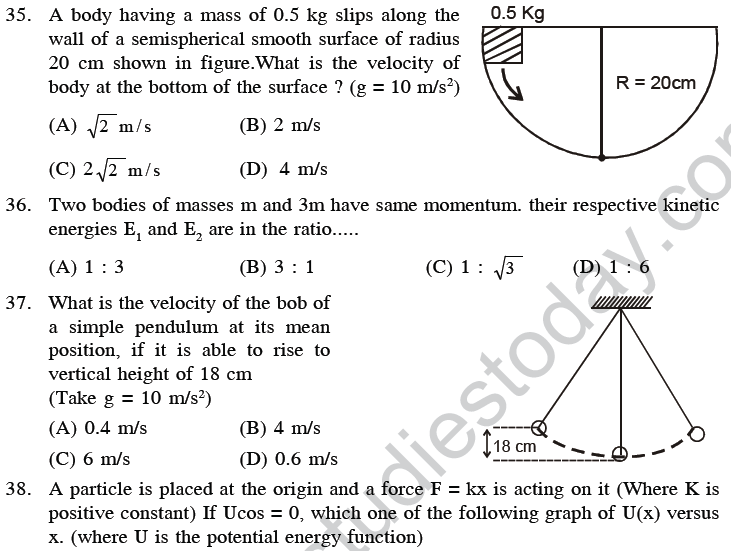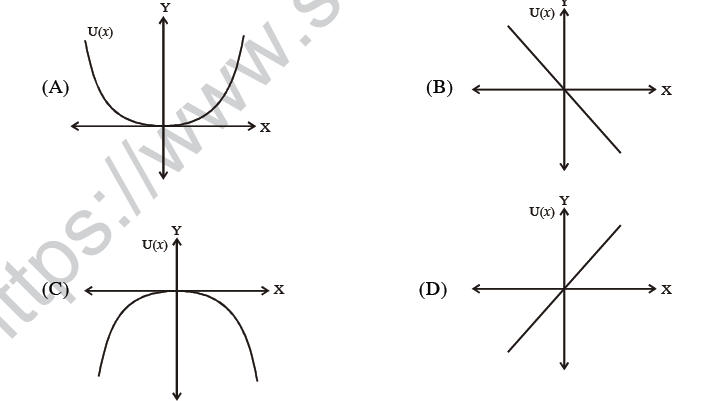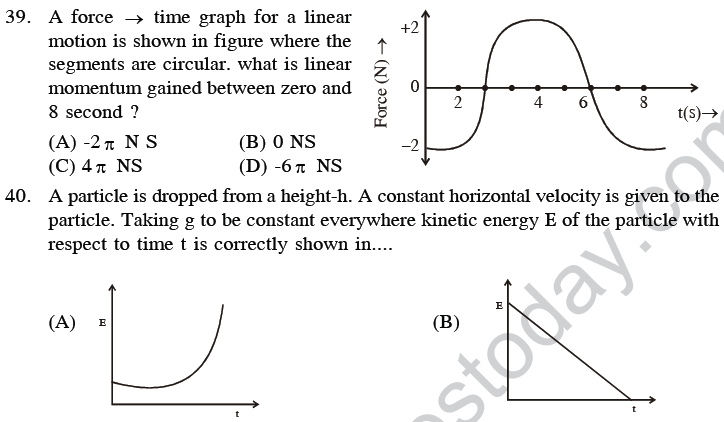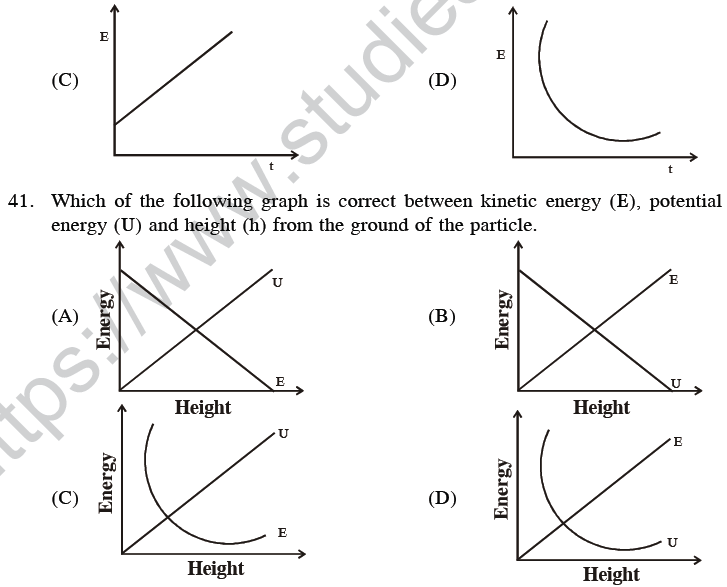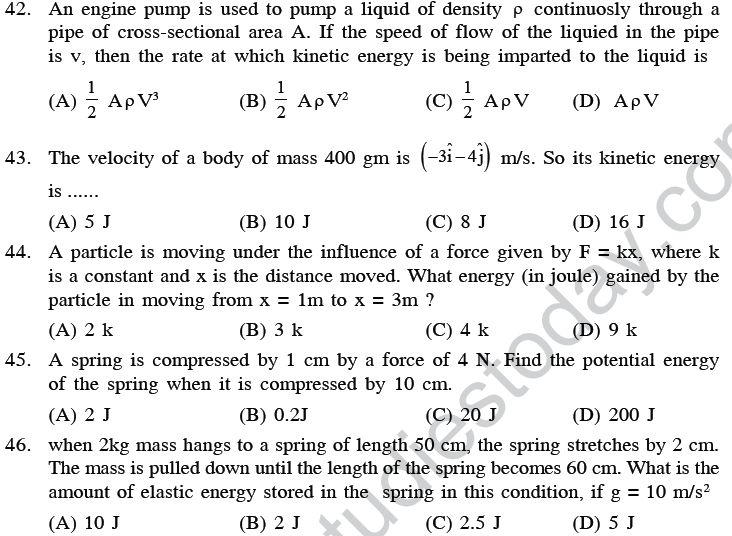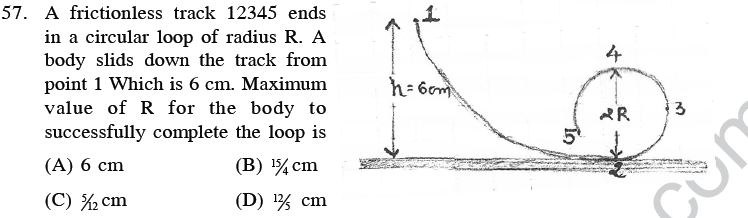58. If the water falls from a dam into a turbine wheel 19.6m below, then the velocity of water at the turbine is ...... ( g = 9.8 m/s2)
(A) 9.8 m/s (B) 19.6 m/s (C) 39.2 m/s (D) 98.0 m/s

59. A bomb of 12 kg divedes in two parts whose ratio of masses is 1:4. If kinetic energy of smaller part is 288 J, then momentum of bigger part in kgm/sec will be
(A) 48 (B) 72 (C) 108 (D) Data is incomplete

60. An ice-cream has a marked value of 700kcal. How many kilo-watt-hour of energy will it deliver to the body as it is digested (J = 4.2 J/cal)
(A) 0.81 kwh (B) 0.90 kwh (C) 1.11 kwh (D) 0.71 kwh62. A bomb of mass 10 kg explodes into 2 pieces of mass 4 kg and 6 kg. The velocity of mass 4 kg is 1.5 m/s, the K.E. of mass 6 kg is ___
(A) 3.84 J (B) 9.6 J (C) 3.00 J (D) 2.5 J

63. A bomb of mass 3.0 kg explodes in air into two pieces of masses 2.0 kg and 1.0 kg. The smaller mass goes at a speed of 80m/s. The total energy imparted to the two fragments is
(A) 1.07 KJ (B) 2.14 KJ (C) 2.4 KJ (D) 4.8 KJ

64. The bob of simple pendulum (mass m and length l) dropped from a horizontal position strike a block of the same mass elastically placed on a horizontal frictionless table. The K.E. of the block will be
(A) 2 mgl (B) mgl /2 (C) mgl (D) zero

65. A gun fires a bullet of mass 40 g with a velocity of 50 m/s. Because of this the gun is pushed back with a velocity of 1 m/s. The mass of the gun is
(A) 1.5 kg (B) 3 kg (C) 2 kg (D) 2.5 kg

66. The decreases in the potential energy of a ball of mass 25 kg which falls from a height of 40 cm is
(A) 968 J (B) 100 J (C) 1980 J (D) 200 J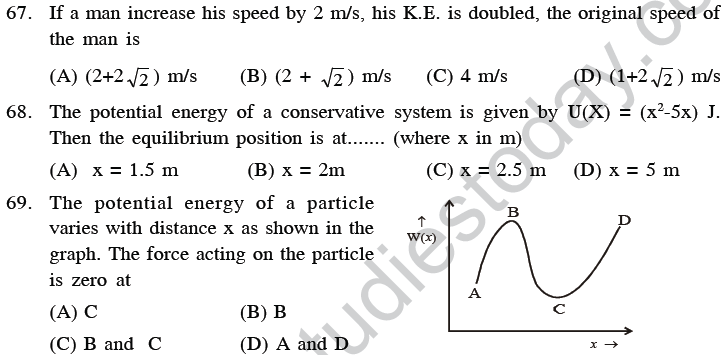70. A nucleus at rest splits into two nuclear parts having same density and radii in the ratio 1:2. Their velocites are in the ratio
(A) 2:1 (B) 4:1 (C) 6:1 (D) 8:1

71. A body of mass 1.5 kg slide down a curved track which is quadrant of a circle of radias 0.75 meter. All the surfaces are frictionless. If the body starts from rest, its speed at the bottom of the track is ____ ( g = 10 m/s2)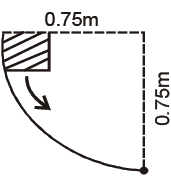(A) 3. 87 m/s (B) 2 m/s
(C) 1.5 m/s (D) 0.387 m/s

72. A single conservative force F(x) acts on a 2.5 kg particle that moves along the x-axis. The potential energy U(x) is given by U(x) = (10 + (x - 4)2) where x is in meter. At x = 6.0m the particle has kinetic energy of 20J. what is the mechanical energy of the system ?
(A) 34 J (B) 45 J (C) 48 J (D) 49 J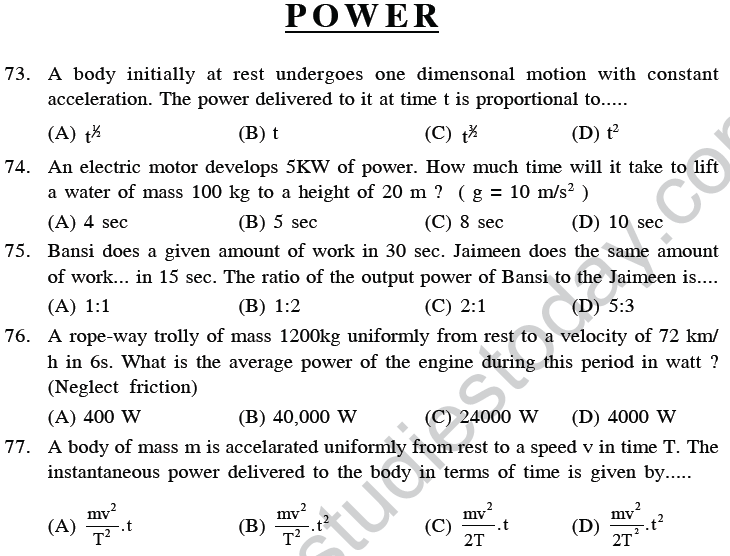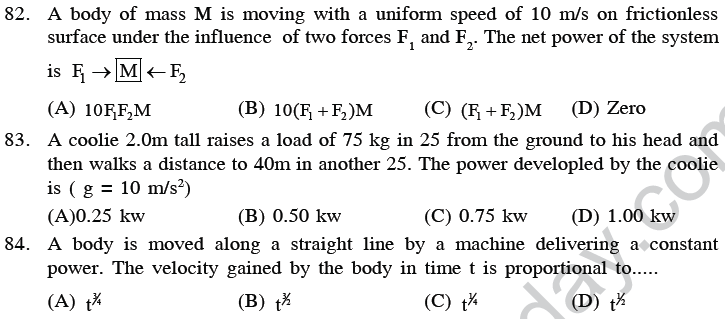89. Three objects A, B and C are kept in a straight line on a frictionless horizontal surface. These have masses m, 2m and m respectivelly. The object A moves towards B with a speed 9 m/s and makes an elastic collision with it. Thereafter, B makes compelety inelastic collision with C. All motion occur on the same straight line. Find final speed of the object C(A) 3 m/s
(B) 4 m/s
(C) 5 m/s (D) 1 m/s

90. Two solid rubber balls P and Q having masses 200 g and 400 g respectively are moving in opposite directions with velocity of P equal to 0.3 m/s. After collision the two balls come to rest, then the velocity of Q is
(A) 0.15 m/s (B) 1.5 m/s (C) -0.15 m/s (D) Zero

91. A sphere collides with another sphere of identical mass. After collision, the two sphere move. The collision is inelastic. Then the angle between the directions of the two spheres is
(A) Different from 90° (B) 90° (C) 0° (D) 45°

92. A ball is allowed to fall from a height 20m . If there is 30% loss of energy due to impact, then after one impact ball will go up to
(A) 18 m (B) 16 m (C) 12 m (D) 14 m

93. If a skater of weight 4 kg has intial speed 40 m/s and 2nd one of weight 6 kg has 6 m/s. After collision, they have speed (couple) 6 m/s. Then the loss in K.E. is ____
(A) 48 J (B) zero (C) 96 J (D) None of
these

94. A metal ball of mass 2 kg moving with a velocity of 36 km/h has a head on collision with a stationary ball of mass 3 kg. If after the collision, the two balls move togather, the loss in kinetic energy due to collision is
(A) 40 J (B) 60 J (C) 100 J (D) 140 J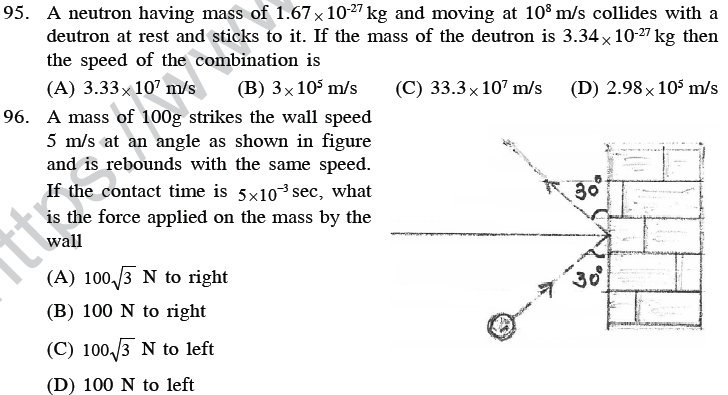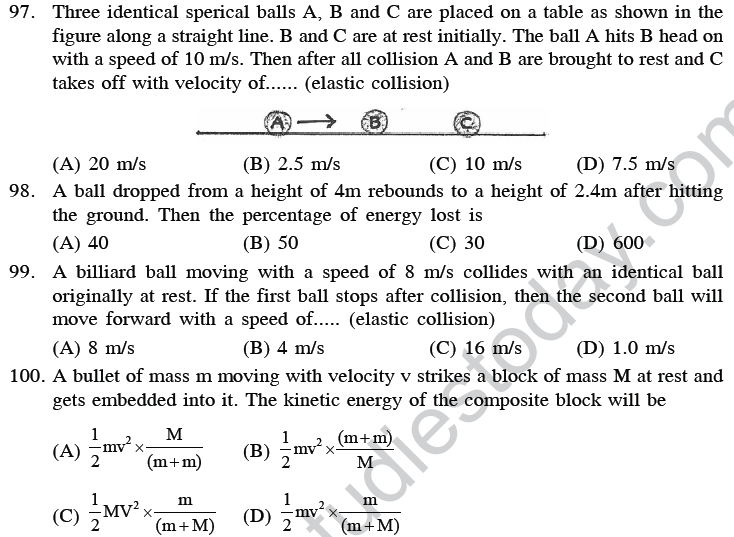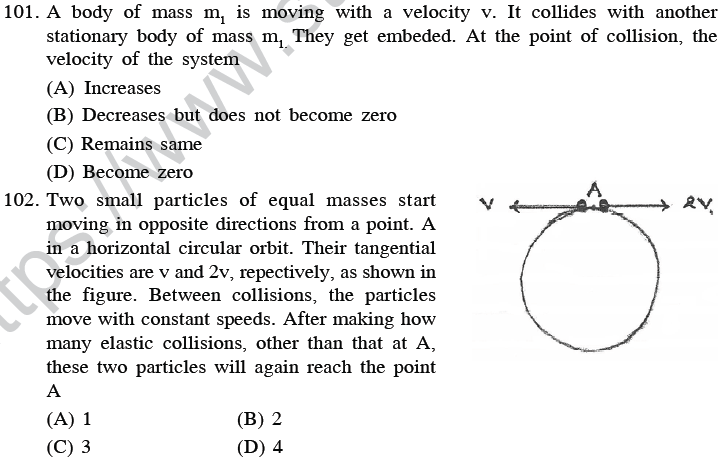103. Four identical balls are lined in a straight grove made on a horizontal frictionless surface as shown. Two similar balls each moving with a velocity v collide elastically with the row of 4 balls from left. What will happen(A) One ball from the right rolls out with a speed 2v and the remaining balls will remain at rest.
(B) Two balls from the right roll out speed v each and the remaining balls will remain statinary.
(C) All the four balls in the row will roll out with speed v/4 each and the two colliding balls will come to rest.
(D) The colliding balls will come to rest and no ball rolls out from right.

(ASSERTION & REASON)
* Assertion and Reason are given in following questions. Each question have four option. One of them is correct it.
(1) If both assertion and reason and the reason is the correct explanation of the Assertion.
(2) If bothe assertion and reason are true but reason is not the correct explanation of the assertion.
(3) If the assertion is true but reason is false.
(4) If the assertion and reason both are fare.

104. Assertion : In the elastic collision between two bodies, the relative speed of the bodies after collision is equal to the relative speed before the collision.
Reason : In the elastic collision the linear momentum of the system is conserved.
(A)1 (B) 2 (C) 3 (D) 4

105. Assertion : When a gas is allowed to expand, work done by gas is positive.
Reason : Force due to gaseous pressure and displacement (of position) on in the same direction.
(A) 1 (B) 2 (C) 3 (D) 4

106. Assertion : A light body and heavy body have same momemtum. Then they also have same kinetic energy.
Reason : Kinetic energy does not depend on mass of the body.
(A) 1 (B) 2 (C) 3 (D) 4

107. Assertion : Mountain roads rarely go straight up the slope.
Reason : slope of mountains are large, therefore, more chances of vehicle to slip from roads.
(A) 1 (B) 2 (C) 3 (D) 4

108. Assertion : The change in kinetic energy of a particle is equal to the work done on it by the net force.
Reason : Change in kinetic energy of particle is equal to the work done only in case of a system of one particle.
(A) 1 (B) 2 (C) 3 (D) 4

109. Assertion : Work done in moving a body over a closed loop is zero for every force in nature.
Reason : Work done does not depend on nature of force.
(A) 1 (B) 2 (C) 3 (D) 4

110. Assertion : A weight lifter does no work in holding the weight up.
Reason : Work done is zero because distance moved is zero.
(A) 1 (B) 2 (C) 3 (D) 4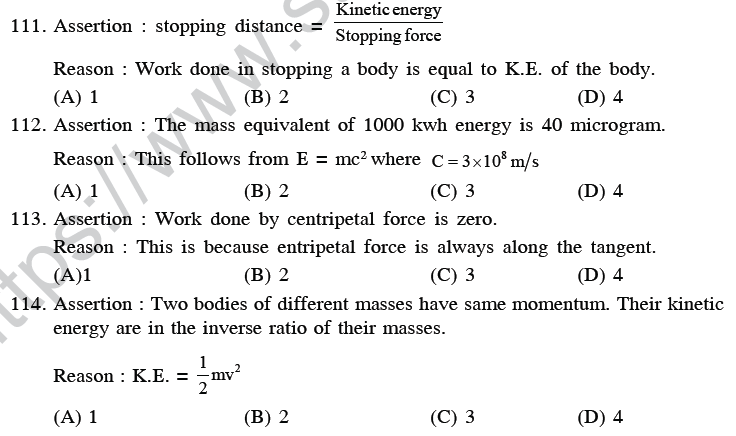115. Assertion : Linear momentum is conserved in both, elastic and inelastic collisions.
Reason : Total energy is conserved in all such collisins.
(A) 1 (B) 2 (C) 3 (D) 4

116. Assertion : Both, a stretched spring and a compressed spring have potential energy.
Reason : Work is done against the restoring force in each case.
(A) 1 (B) 2 (C) 3 (D) 4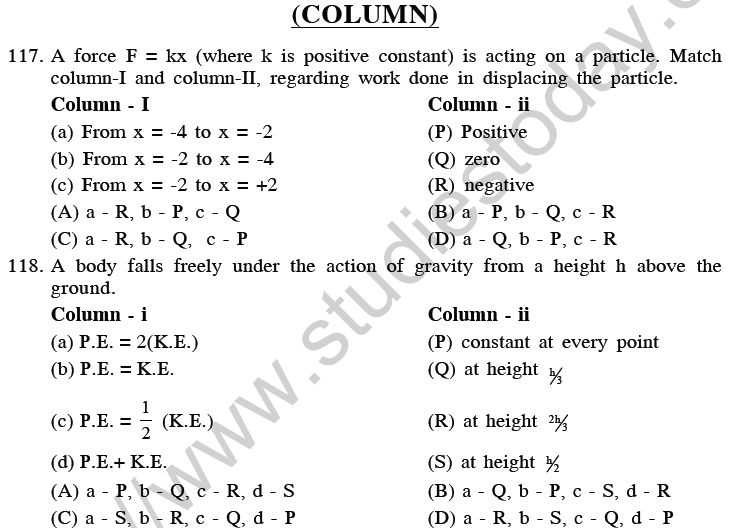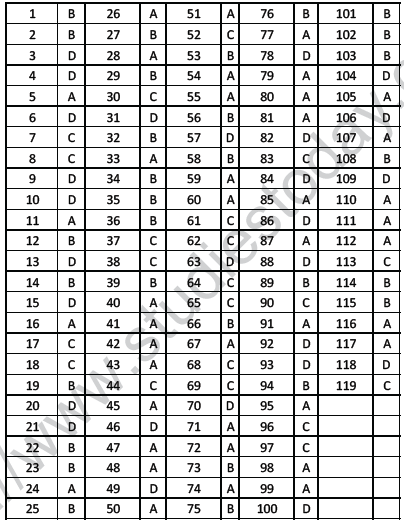## Tags:

Click for more Physics Study Material
 NEET UG Physics Laws of Motion MCQs NEET UG Physics Electromagnetic Waves MCQs NEET UG Physics Thermodynamics MCQs NEET UG Physics Atom and Nucleus MCQs NEET UG Physics Magnetic Effects of Electric Current MCQs NEET UG Physics Electromagnetic Induction MCQs NEET UG Physics Work Energy MCQs NEET UG Physics Communication System MCQs NEET UG Physics Oscillations and Waves MCQs NEET UG Physics Gravitational MCQs NEET Physics Digital Electronics and Logic Gates MCQs NEET UG Physics Current Electricity MCQs NEET UG Physics Optics MCQs NEET UG Physics Kinetics and Measurement MCQs NEET UG Physics Dual Nature of Matter MCQs NEET UG Physics Properties of Liquid of Solid MCQs NEET UG Physics Kinetics MCQs NEET UG Physics Experiments MCQs NEET UG Physics Rational Motion MCQs NEET UG Physics Kinetic Theory of Gases MCQs NEET UG Physics Electrostatics MCQs NEET UG Physics Semiconductor Electronics MCQs

## Latest NCERT & CBSE News

Read the latest news and announcements from NCERT and CBSE below. Important updates relating to your studies which will help you to keep yourself updated with latest happenings in school level education. Keep yourself updated with all latest news and also read articles from teachers which will help you to improve your studies, increase motivation level and promote faster learning

### CBSE Class 10 Revised Syllabus

Last year CBSE had to reduce the syllabus because of the pandemic situation but it was not very effective because there were no examinations. This year to avoid any confusion and conflict, CBSE has decided to reduce the syllabus into term 1 and term 2. 50 percent of...

### Score well in Class 12 English Boards Exam

12th Board exams are an important part of students' lives. The marks obtained in the board exam decide the college in which one can study. In class 12 the syllabus of each and every subject increases vastly and it is difficult to cover up every point. In English also...

### MCQ Question based CBSE examination

For 2021-22 CBSE has launched MCQ question-based examination for Term 1 & Term 2 board examinations. The entire syllabus has been divided into two parts each including 50% of the entire syllabus. To score well, students must practice as per the new CBSE term-wise...

### How To Solve Unseen Passages In English

Unseen passages may contain one or many paragraphs. This is one of the important yet easy parts for a student to get marks. Students should thoroughly study and understand the passage to answer the related questions. The unseen passages are there just to test the...

×